Get inspired by the success stories of our students in IIT JAM MS, ISI  MStat, CMI MSc DS.  Learn More

# ISI MStat PSB 2014 Problem 2 | Properties of a FunctionThis is a very beautiful sample problem from ISI MStat PSB 2014 Problem 2 based on the use and properties of a function . Let's give it a try !!

## Problem- ISI MStat PSB 2014 Problem 2

Let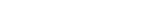and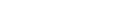be real numbers such
that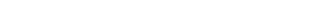Show that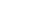and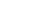for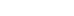### Prerequisites

Differentiability

Mod function

continuity

## Solution :

Let ,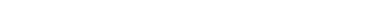Then ,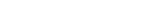is not differentiable at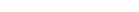---(1)

As we know the function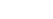is not differentiable at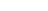.

Again we have ,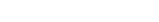it also not differentiable at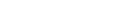----(2)

Hence from (1) we get f has m non-differentiable points and from (2) we get f has n non-differentiable points , which is possible only when m and n are equal .

And also the points where f is not differentiable must be same in both (1) and (2) .

As we have the restriction thatand.

So , we havefor.

## Food For Thought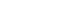Let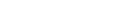be a continuous and differentiable on (a,b) . Suppose that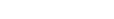for all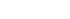for some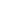Then prove that there exists unique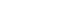such that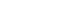## Subscribe to Cheenta at Youtube

This is a very beautiful sample problem from ISI MStat PSB 2014 Problem 2 based on the use and properties of a function . Let's give it a try !!

## Problem- ISI MStat PSB 2014 Problem 2

Letandbe real numbers such
thatShow thatandfor### Prerequisites

Differentiability

Mod function

continuity

## Solution :

Let ,Then ,is not differentiable at---(1)

As we know the functionis not differentiable at.

Again we have ,it also not differentiable at----(2)

Hence from (1) we get f has m non-differentiable points and from (2) we get f has n non-differentiable points , which is possible only when m and n are equal .

And also the points where f is not differentiable must be same in both (1) and (2) .

As we have the restriction thatand.

So , we havefor.

## Food For ThoughtLetbe a continuous and differentiable on (a,b) . Suppose thatfor allfor someThen prove that there exists uniquesuch that## Subscribe to Cheenta at Youtube

This site uses Akismet to reduce spam. Learn how your comment data is processed.

### One comment on “ISI MStat PSB 2014 Problem 2 | Properties of a Function”

1.abhishek sinha says:

This problem has an absolutely elementary solution. For any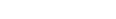, the given condition implies that: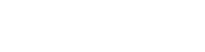i.e.,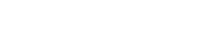If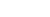, this linear equation has only one solution. But the above equation should be valid for any of the infinitely many. Hence we must haveand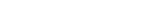.\\
Next, if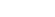, assume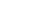, and choose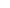such that. The given condition implies that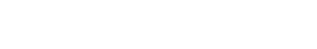This, coupled with the previous result, implies that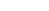, which is a contradiction. Hence we have. Proceeding similarly, it follows that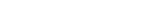.

### Knowledge Partner